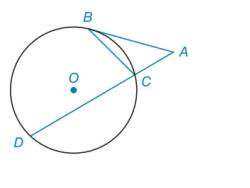Chapter 6.2, Problem 23E### Elementary Geometry for College St...

6th Edition
Daniel C. Alexander + 1 other
ISBN: 9781285195698

#### Solutions

Chapter
Section### Elementary Geometry for College St...

6th Edition
Daniel C. Alexander + 1 other
ISBN: 9781285195698
Textbook Problem
1 views

# In Exercises 23 to 25 , complete a paragraph proof. Given: Tangent A B ¯ to ⊙ ο at point B m ∠ A = m ∠ B Prove: m B D ⌢ = 2 ⋅ m B C ⌢To determine

To find:

The prove mBD=2mBC.

Explanation

Calculation:

Given,

mA=mB.

To prove: mBD=2mBC.

Since, Tangent AB¯ to ο at point B.

A is a tangent-secant angle, mA=mBDmBC2.

B has the vertex on the circle and is a chord-tangent angle, mB=mBC2.

Given,

mA=mB

Substitute the values of mA and mB in mA=mB

### Still sussing out bartleby?

Check out a sample textbook solution.

See a sample solution

#### The Solution to Your Study Problems

Bartleby provides explanations to thousands of textbook problems written by our experts, many with advanced degrees!

Get Started